# Python OpenCV 图像处理之傅里叶变换，取经之旅第 52 篇Python OpenCV 365 天学习计划，与橡皮擦一起进入图像领域吧。本篇博客是这个系列的第 52 篇。

## 学在前面

• 使用高通滤波器之后，会保留高频信息，增强图像细节，例如边界增强；

• 使用低通滤波器之后，会保留低频信息，边界模糊。

## 傅里叶变换应用

### Numpy 实现傅里叶变换

fft2(a, s=None, axes=(-2, -1), norm=None)

• a：输入图像；

• s：整数序列，输出数组的大小；

• axex：整数序列，用于计算 FFT 的可选轴；

• norm：规范化模式。

import cv2 as cvimport numpy as npfrom matplotlib import pyplot as pltimg = cv.imread('test.jpg', 0)# 快速傅里叶变换算法得到频率分布，将空间域转化为频率域f = np.fft.fft2(img)# 默认结果中心点位置是在左上角,通过下述代码将中心点转移到中间位置# 将低频部分移动到图像中心fshift = np.fft.fftshift(f)# fft 结果是复数, 其绝对值结果是振幅result = 20*np.log(np.abs(fshift))plt.subplot(121)plt.imshow(img, cmap='gray')plt.title('original')plt.axis('off')plt.subplot(122)plt.imshow(result, cmap='gray')plt.title('result')plt.axis('off')plt.show()

import cv2 as cvimport numpy as npfrom matplotlib import pyplot as plt# 生成一个图片src = np.ones((5, 5), dtype=np.uint8)*100print(src)print(src.shape)f = np.fft.fft2(src,(7,7))print(f.shape)print(f)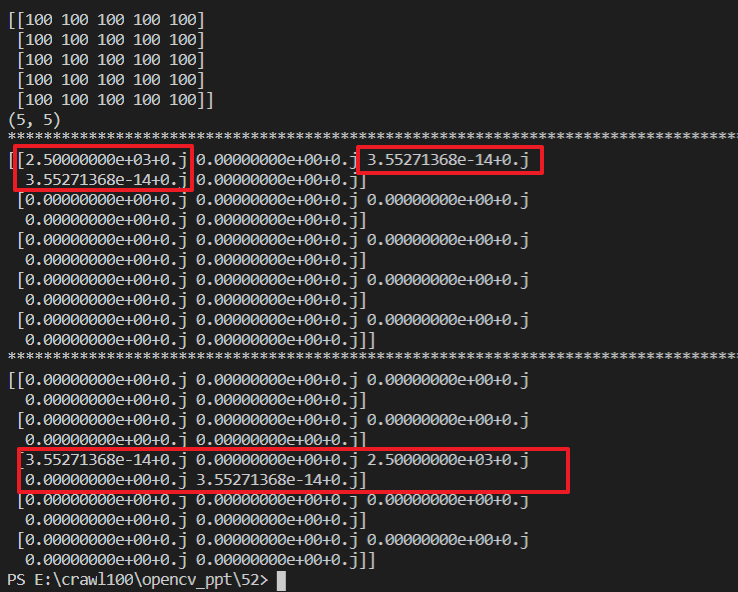fimg = np.log(np.abs(fshift))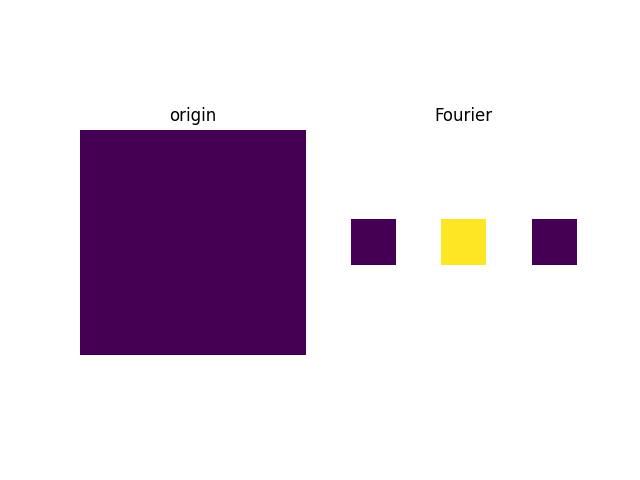import cv2 as cvimport numpy as npfrom matplotlib import pyplot as plt# 生成一个图片# src = np.ones((5, 5), dtype=np.uint8)*100src = cv.imread("./test.jpg", 0)print("*"*100)print(src)print(src.shape)f = np.fft.fft2(src)print("*"*100)print(f)fshift = np.fft.fftshift(f)print("*"*100)print(fshift)# 将复数转为浮点数进行傅里叶频谱图显示fimg = 20*np.log(np.abs(fshift))print(fimg)# 图像显示plt.subplot(121), plt.imshow(src, "gray"), plt.title('origin')plt.axis('off')plt.subplot(122), plt.imshow(fimg, "gray"), plt.title('Fourier')plt.axis('off')plt.show()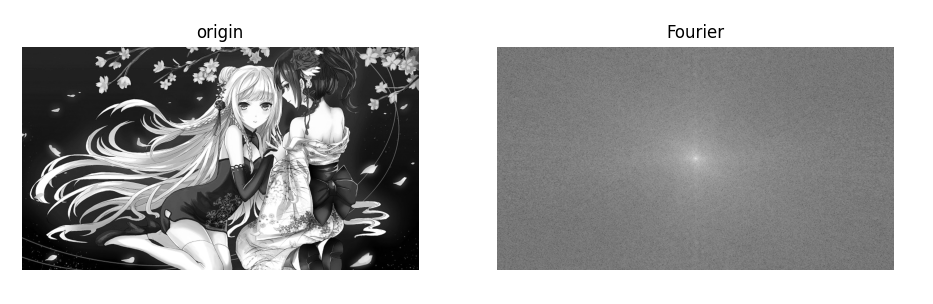# 实现图像逆傅里叶变换，返回一个复数数组np.fft.ifft2(a, s=None, axes=(-2, -1), norm=None)

np.fft.ifftshift

# fftshit()函数的逆函数，它将频谱图像的中心低频部分移动至左上角np.fft.ifftshift(x, axes=None)

import cv2 as cvimport numpy as npfrom matplotlib import pyplot as plt# 生成一个图片# src = np.ones((5, 5), dtype=np.uint8)*100src = cv.imread("./test.jpg", 0)print("*"*100)print(src)print(src.shape)f = np.fft.fft2(src)print("*"*100)print(f)fshift = np.fft.fftshift(f)print("*"*100)print(fshift)# 将复数转为浮点数进行傅里叶频谱图显示fimg = np.log(np.abs(fshift))print(fimg)# 逆傅里叶变换ifshift = np.fft.ifftshift(fshift)# 将复数转为浮点数进行傅里叶频谱图显示ifimg = np.log(np.abs(ifshift))if_img = np.fft.ifft2(ifshift)origin_img = np.abs(if_img)# 图像显示plt.subplot(221), plt.imshow(src, "gray"), plt.title('origin')plt.axis('off')plt.subplot(222), plt.imshow(fimg, "gray"), plt.title('fourier_img')plt.axis('off')plt.subplot(223), plt.imshow(origin_img, "gray"), plt.title('origin_img')plt.axis('off')plt.subplot(224), plt.imshow(ifimg, "gray"), plt.title('ifimg')plt.axis('off')plt.show()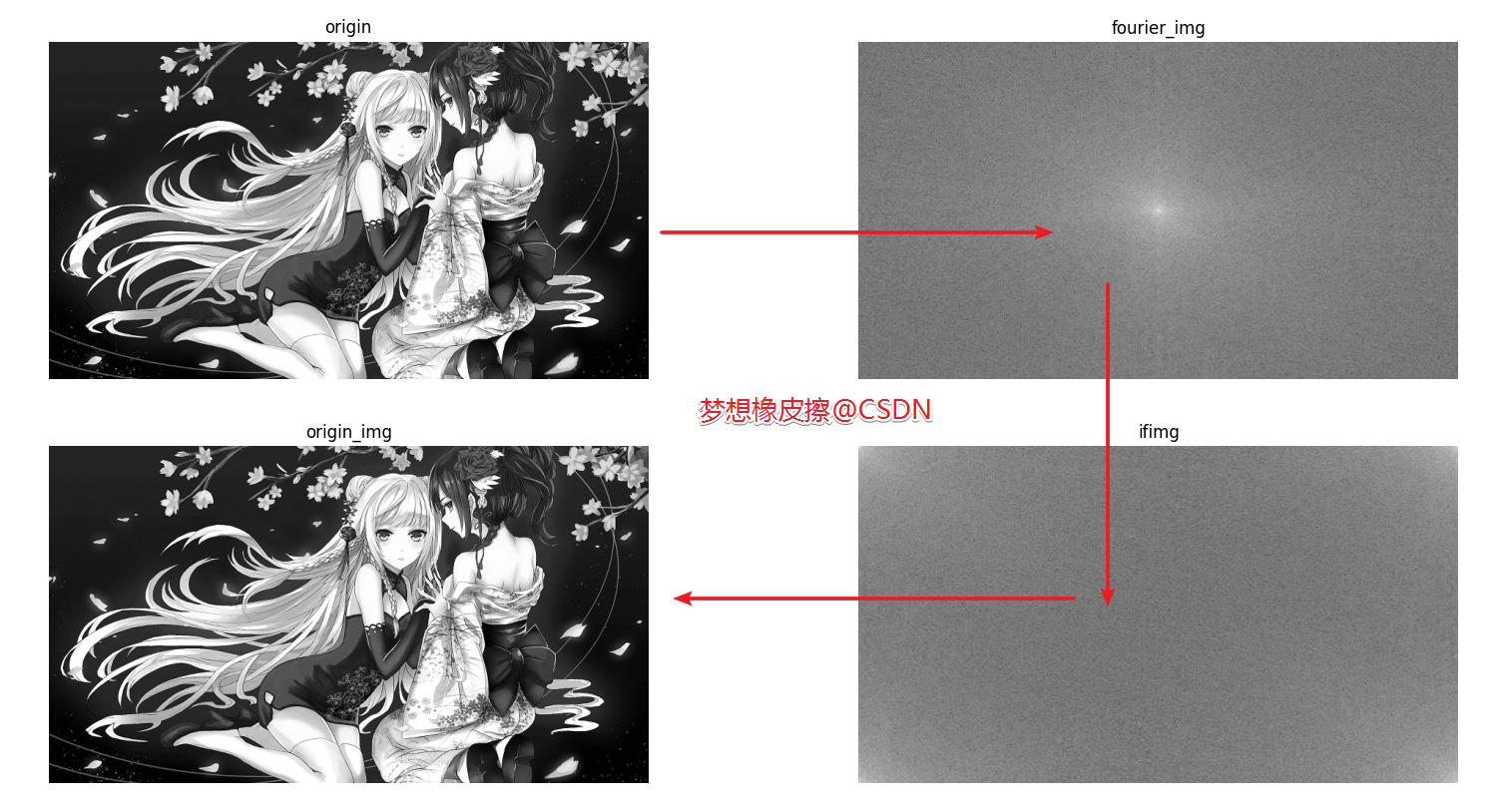import cv2 as cvimport numpy as npfrom matplotlib import pyplot as plt# 实现傅里叶变换的低通滤波src = cv.imread("./test.jpg", 0)f = np.fft.fft2(src)fshift = np.fft.fftshift(f)rows, cols = src.shapecrow, ccol = rows//2, cols//2# 制定掩模，大小和图像一样，np.zeros初始化mask = np.zeros((rows, cols), np.uint8)# 使中心位置，上下左右距离30，置为1mask[crow-30:crow+30, ccol-30:ccol+30] = 1# 掩模与 DFT 后结果相乘只保留出中间区域fshift = fshift*mask# 逆傅里叶变换ifshift = np.fft.ifftshift(fshift)# 将复数转为浮点数进行傅里叶频谱图显示ifimg = np.fft.ifft2(ifshift)dft_img = np.abs(ifimg)# 图像显示plt.subplot(121), plt.imshow(src, "gray"), plt.title('origin')plt.axis('off')plt.subplot(122), plt.imshow(dft_img, "gray"), plt.title('dft_img')plt.axis('off')plt.show()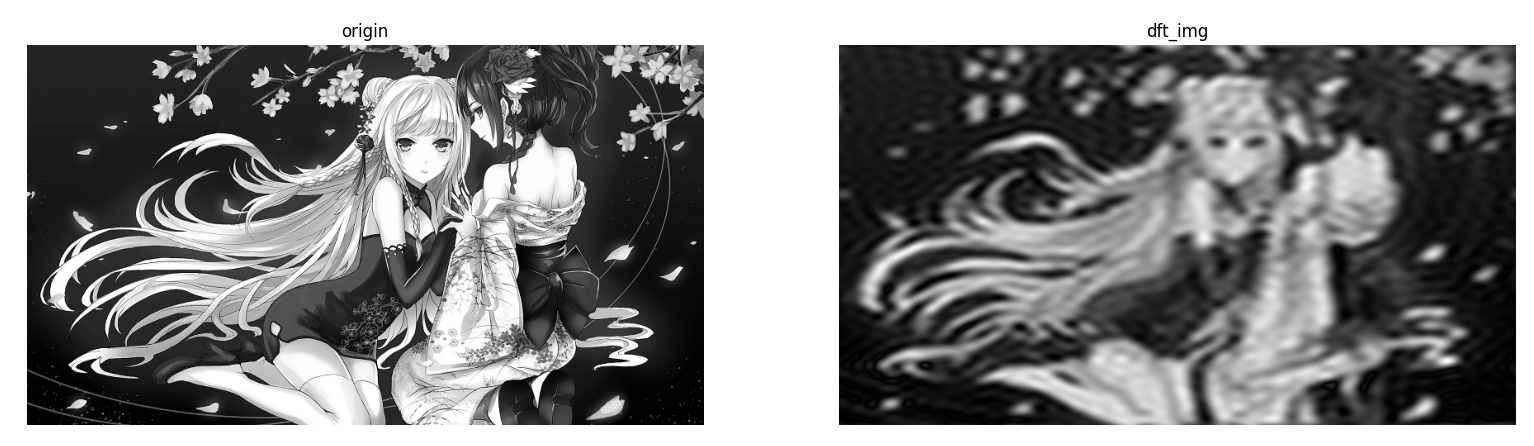mask = np.ones((rows, cols), np.uint8)mask[crow-30:crow+30, ccol-30:ccol+30] = 0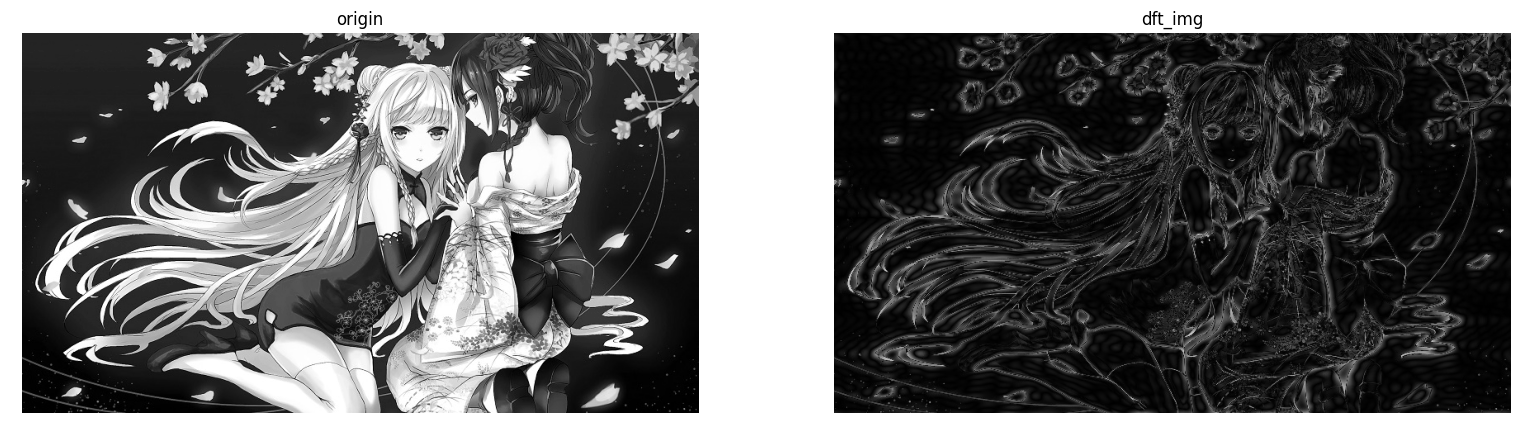### OpenCV 实现傅里叶变换

OpenCV 实现傅里叶变换与 numpy 基本一致，核心差异在函数的使用上，具体区别如下：Opencv 中主要通过 cv2.difcv2.idif（傅里叶逆变换），在输入图像之前需要先转换成把图像从 np.uint8 转换为 np.float32 格式，其得到的结果中频率为 0 的部分会在左上角，要转换到中心位置，通过 shift 变换来实现，与 numpy 一致，cv2.dif 返回的结果是双通道的（实部，虚部），还需要转换成图像格式才能展示。

dst = cv.dft(src[, dst[, flags[, nonzeroRows]]])

• src：输入图像，要求 np.float32 格式；

• dst：输出图像，双通道（实部+虚部），大小和类型取决于第三个参数 flags

• flags：表示转换标记，默认为 0，存在多种取值，参见后文；

• nonzeroRows：默认为 0，暂时不考虑。

flags 取值如下：

• DFT_INVERSE：用一维或二维逆变换取代默认的正向变换；

• DFT_SCALE： 缩放比例标识符，根据数据元素个数平均求出其缩放结果，如有 N 个元素，则输出结果以 1/N 缩放输出，常与 DFT_INVERSE 搭配使用；

• DFT_ROWS：对输入矩阵的每行进行正向或反向的傅里叶变换；此标识符可在处理多种适量的的时候用于减小资源的开销，这些处理常常是三维或高维变换等复杂操作；

• DFT_COMPLEX_OUTPUT：对一维或二维的实数数组进行正向变换，这样的结果虽然是复数阵列，但拥有复数的共轭对称性（CCS），可以以一个和原数组尺寸大小相同的实数数组进行填充，这是最快的选择也是函数默认的方法。你可能想要得到一个全尺寸的复数数组（像简单光谱分析等等），通过设置标志位可以使函数生成一个全尺寸的复数输出数组；

• DFT_REAL_OUTPUT：对一维二维复数数组进行逆向变换，这样的结果通常是一个尺寸相同的复数矩阵，但是如果输入矩阵有复数的共轭对称性（比如是一个带有 DFT_COMPLEX_OUTPUT标识符的正变换结果），便会输出实数矩阵。

• DFT_COMPLEX_OUTPUT：得到一个复数形式的矩阵；

• DFT_REAL_OUTPUT：只输出复数的实部；

• DFT_INVERSE：进行傅里叶逆变换；

• DFT_SCALE：是否除以 MxN （M 行 N 列的图片，共有有 MxN 个像素点）；

• DFT_ROWS：输入矩阵的每一行进行傅里叶变换或者逆变换。

cv2.magnitude 函数原型：cv2.magnitude(x, y)

• x 表示浮点型 X 坐标值，即实部

• y 表示浮点型 Y 坐标值，即虚部

import cv2 as cvimport numpy as npimport matplotlib.pyplot as pltplt.rcParams['font.sans-serif'] = ['SimHei']src = cv.imread("test.jpg", 0)# OpneCV傅里叶变换函数# 需要将图像进行一次float转换result = cv.dft(np.float32(src), flags=cv.DFT_COMPLEX_OUTPUT)# 将频谱低频从左上角移动至中心位置dft_shift = np.fft.fftshift(result)# 频谱图像双通道复数转换为 0-255 区间result1 = 20 * np.log(cv.magnitude(dft_shift[:, :, 0], dft_shift[:, :, 1]))# 图像显示plt.subplot(121), plt.imshow(src, 'gray'), plt.title('原图像')plt.axis('off')plt.subplot(122), plt.imshow(result1, 'gray'), plt.title('傅里叶变换')plt.axis('off')plt.show()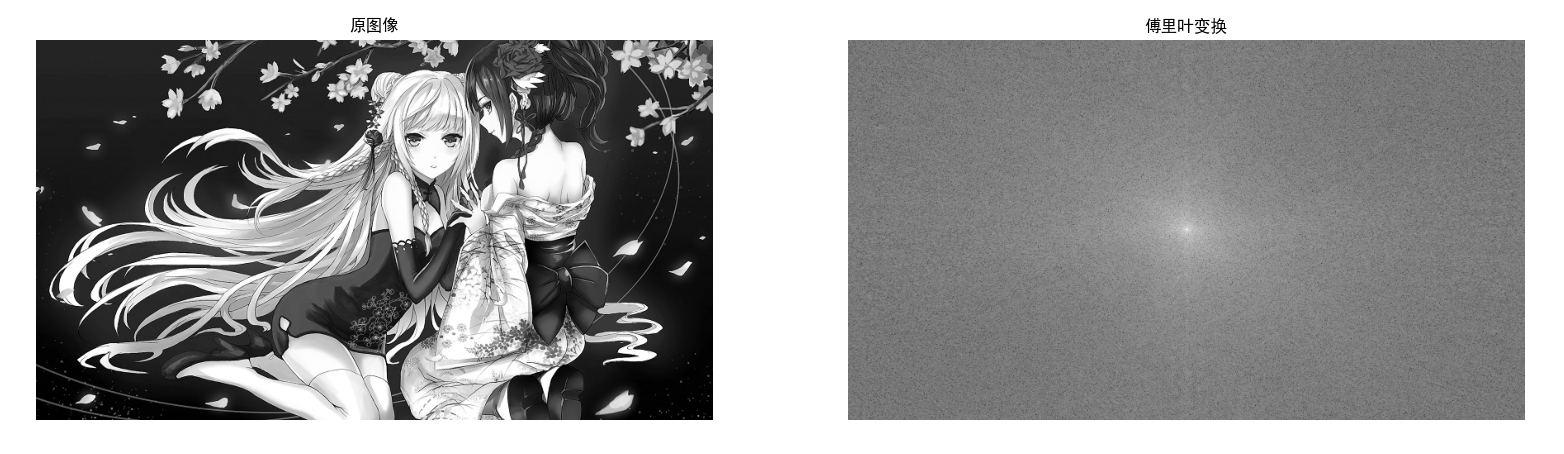import cv2 as cvimport numpy as npimport matplotlib.pyplot as pltplt.rcParams['font.sans-serif'] = ['SimHei']src = cv.imread("test.jpg", 0)# OpneCV傅里叶变换函数# 需要将图像进行一次float转换result = cv.dft(np.float32(src), flags=cv.DFT_COMPLEX_OUTPUT)# 将频谱低频从左上角移动至中心位置dft_shift = np.fft.fftshift(result)# 频谱图像双通道复数转换为 0-255 区间result = 20 * np.log(cv.magnitude(dft_shift[:, :, 0], dft_shift[:, :, 1]))rows, cols = src.shapecrow, ccol = rows//2, cols//2mask = np.zeros((rows, cols, 2), np.uint8)mask[int(crow-30):int(crow+30), int(ccol-30):int(ccol+30)] = 1#  LPF（低通滤波）fshift = dft_shift*maskf_ishift = np.fft.ifftshift(fshift)img_back = cv.idft(f_ishift)img_back = cv.magnitude(img_back[:, :, 0], img_back[:, :, 1])# 图像显示plt.subplot(131), plt.imshow(src, 'gray'), plt.title('原图像')plt.axis('off')plt.subplot(132), plt.imshow(result, 'gray'), plt.title('傅里叶变换')plt.axis('off')plt.subplot(133), plt.imshow(img_back, 'gray'), plt.title('低通滤波之后的图像')plt.axis('off')plt.show()## 橡皮擦的小节

HPF（高通滤波），LPF（低通滤波）希望今天的 1 个小时（貌似不太够）你有所收获，我们下篇博客见~### 梦想橡皮擦

6 年产品经理+教学经验，3 年互联网项目管理经验； 互联网资深爱好者； 沉迷各种技术无法自拔，导致年龄被困在 25 岁； CSDN 爬虫 100 例作者。 个人公众号“梦想橡皮擦”。

## 评论